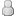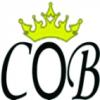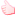Thread Rating:
• 1 Votes - 5 Average
• 1
• 2
• 3
• 4
• 5
 Update Harga H2H CeoBe Tgl 20-01-2016 Author Message
IVAN_PURNAMAJuraganPosts: 20
Joined: Oct 2013
Reputation: 0
Thanks: 0
Given 1 thank(s) in 1 post(s)
 Post: #1Update Harga H2H CeoBe Tgl 20-01-2016 INFO HARGA H2H CEOBE: 20-01-2016 *TSEL NAS BT5 = 5500 BT10 = 10400 BT20 = 19875 BT25 = 24325 BT50 = 48550 BT100 = 94900 *ISAT NAS BI5 = 5475 BI10 = 10475 BI20 = 19950 BI25 = 24800 BI30 = 29700 BI50 = 49350 BI100 = 97800 *XL + AXIS NAS BX5 = 5500 BX10 = 10275 BX25 = 24475 BX50 = 48850 BX100 = 97700 *THREE NAS TH5 = 4965 TH10 = 9875 TH20 = 19750 TH30 = 29575 TH50 = 49250 TH100 = 98350 *SMARTFREN NAS BSF5 = 5025 BSF10 = 9925 BSF20 = 19675 BSF25 = 24550 BSF50 = 48900 BSF100 = 97200 *ESIA NAS BE5 = 3800 BE10 = 7500 BE25 = 18650 BE50 = 37200 BE100 = 74200 Berminat, Hub YM CS Kami: cobpulsa_cs Nama Server: CeoBe RELOAD ID TTS: 213018e03029 Owner: Ivan Purnama Alamat: Jalan Mangga 1/E-200 Pondok Candra Indah, Waru, Sidoarjo - JATIM No HP: 085105002357 / 085648559969 Pin BB: 2102760B Website H2H: http://www.cobpulsa.com/p/harga-h2h-hth.html
01-20-2016 05:26 AM
 « Next Oldest | Next Newest »

Forum Jump:

User(s) browsing this thread: 1 Guest(s)